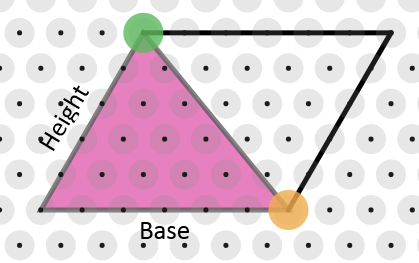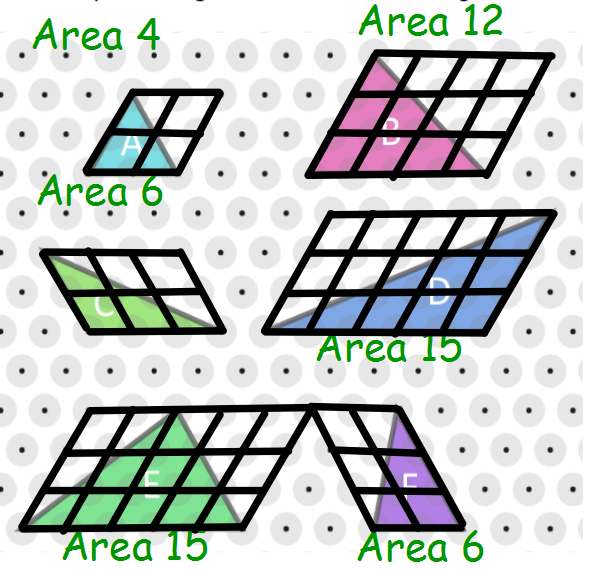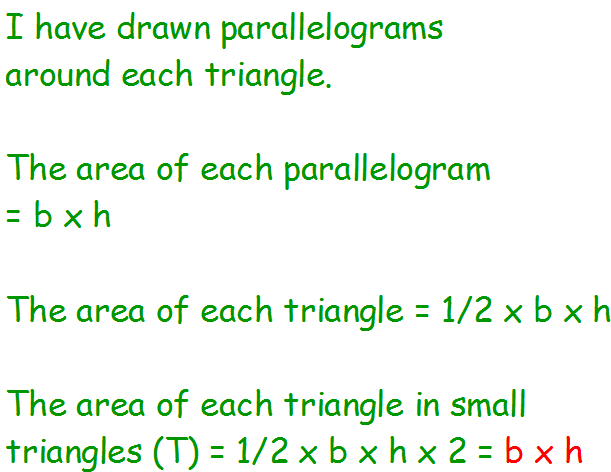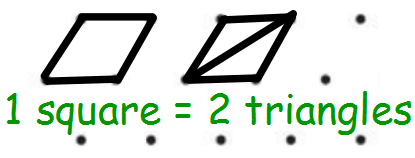#### You may also like### Kissing Triangles

Determine the total shaded area of the 'kissing triangles'.### Isosceles

Prove that a triangle with sides of length 5, 5 and 6 has the same area as a triangle with sides of length 5, 5 and 8. Find other pairs of non-congruent isosceles triangles which have equal areas.### Linkage

Four rods, two of length a and two of length b, are linked to form a kite. The linkage is moveable so that the angles change. What is the maximum area of the kite?

# More Isometric Areas

##### Age 11 to 14Challenge Level
Matt from Swindon Academy in the UK, Ambrose, Swiss and Piyush from West Island School in Hong Kong and Pete and Neha from International School of Lausanne in Switzerland found a formula for finding the areas of the triangles. Piyush said:

Area of a triangle on isometric paper = Whole number side length $\times$ other whole number side length

Pete and Neha, Matt and Ambrose used parallelograms to explain why this works. This is Ambrose's work:
For every triangle with at least two whole number sides, you can always form a parallelogram by putting 2 triangles together.Then, from the task, “Isometric Areas”, we know that the area of a parallelogram in “$T$” is $base\times height\times2$.

For the area of a triangle, we can divide this by $2$

So the formula is: $base\times height$.

Matt thought about using small parallelograms, or 'squares', as units:Swiss also thought about using little parallelograms as 1 unit of area:
To find the area of a normal triangle, the formula is b $\times$ h $\div$ 2 but since we're trying to find number of Triangles, you don't need to divide by 2 because 2$T$=1 unit of area.